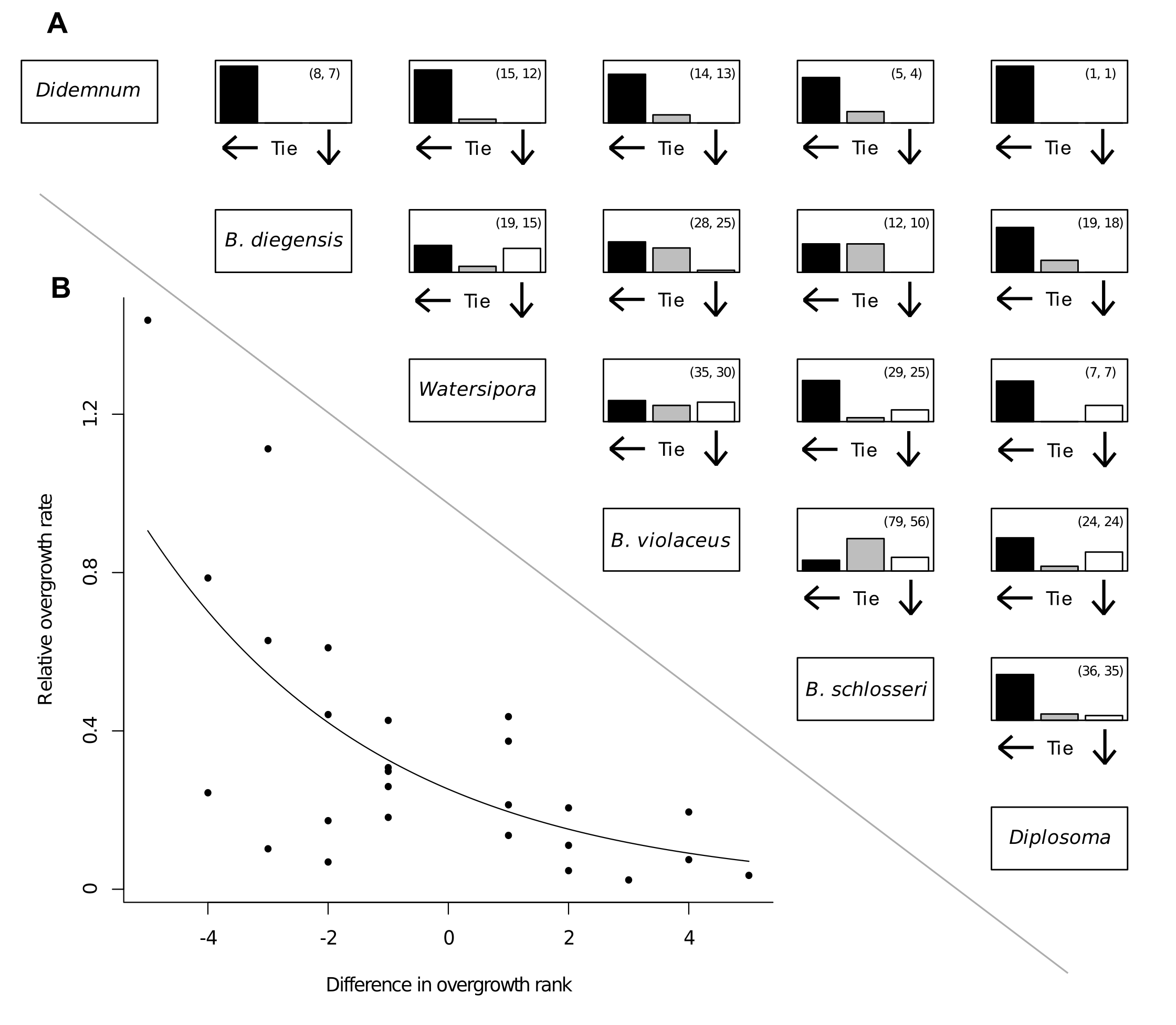### Kyle F. Edwards and John J. Stachowicz. 2010. Multivariate trade-offs, succession, and phenological differentiation in a guild of colonial invertebrates. Ecology 91:3146–3152.

Appendix D. Overgrowth interactions.FIG. D1. (A) summarizes the outcome of all measured interactions. Species are organized from the upper left to the lower right in order of ranked overgrowth ability. Each barplot represents one pair of species, with the black bar showing the proportion of encounters won by the species to the left, the grey bar showing the proportion of ties, and the white bar showing the propotion of encounters won by the species at bottom. In parentheses the first number is total encounters measured, and the second number is total number of unique pairs of colonies measured. (B) shows relative overgrowth rate as a function of difference in overgrowth rank. Each point represents the average relative overgrowth rate for a pair of species. Species are ranked from 1 (best overgrower) to 6 (worst overgrower), so a negative number on the X-axis indicates a better competitor overgrowing a worse competitor. The curve represents a generalized linear model with log link and gamma error; the exponential slope parameter is significantly different from zero (Wald t-test, t = -5.5, df = 24, P < 0.001).

[Back to E091-220]